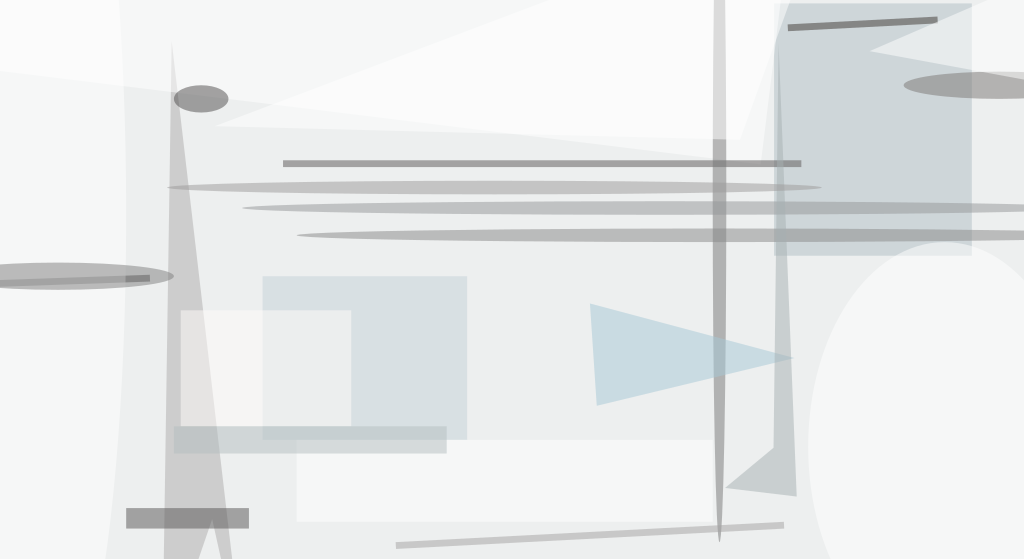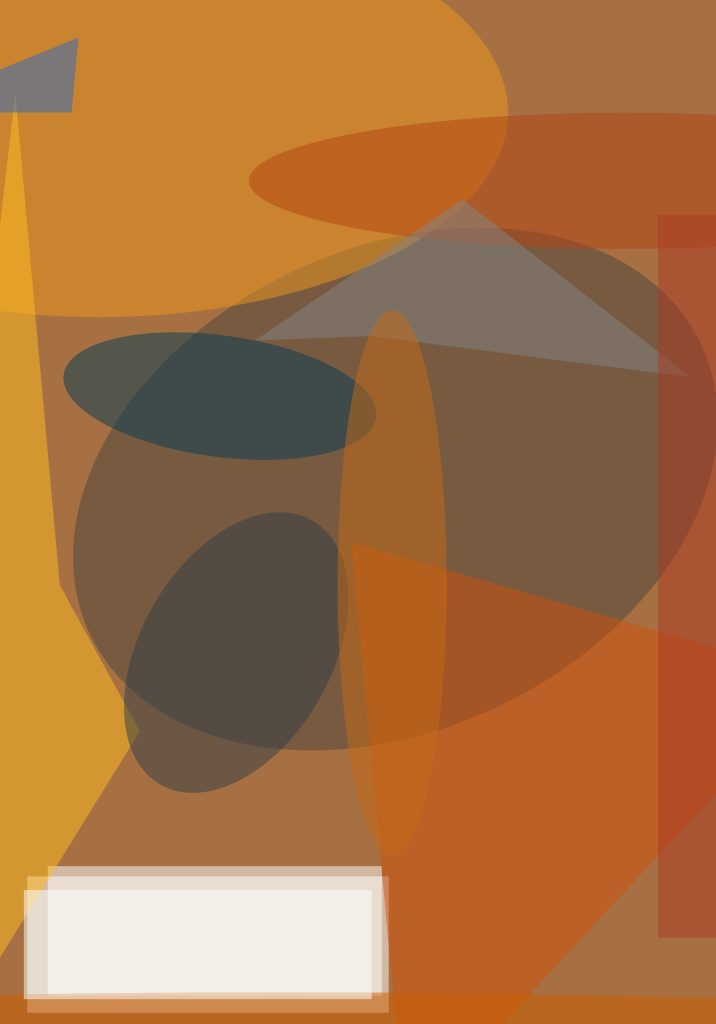An improved technique of limited-precision arithmetic has been devised for use in convolutional filtering of image data prior to conversion of the data to binary form. Both limited-precision arithmetic and conversion to binary form reduce the volume of data, thereby contributing to processing speed and reducing the sizes of circuits needed to perform, and store the results of, intermediate stages of processing. Applications in which these techniques are or could be helpful include robotic vision, recognizing and tracking moving targets, stabilizing images in hand-held video cameras, and general enhancement of images.This Circuit Generates a Saturated Four-Bit Output in response to a six-bit input. The exclusive-OR gates examine the number of high-order bits to be eliminated, plus one, to determine whether they are already all alike. If so, the input quantity is deemed to be within the saturation range, and is itself gated through the multiplexer. If not, a maximum positive or negative quantity is derived from the sign bit and gated through the multiplexer. This circuit implements 2's-complement encoding of negative numbers. The improved technique of limited-precision arithmetic involves the use of saturation — the substitution of a specified datum for a datum of greater magnitude; this is fundamentally different from the conventional practice of truncation or rounding-off of least-significant bits. This technique was conceived especially for use in processing digitized pixel image intensities by convolutional 3 × 3 (or by equivalent combinations of 1 × 3 and 3 × 1 filters) that perform Gaussian, Laplacian, or Laplacian-of-Gaussian (LoG) operations on pixels in 3 × 3 neighborhoods. (Most LoG operations for neighborhoods larger than 3 × 3 can be composed of series of 3 × 3 operations: for example, the LoG for a 9 × 9 neighborhood can be computed by performing four successive 3 × 3 LoG operations.) These filters are typically applied to improve the reliability and consistency of mapping of pixel intensities to binary representation. A Laplacian filter emphasizes edges and texture, while a Gaussian filter smooths out noise.

The preferability of the present saturation-based technique over the conventional truncation-based technique arises in the treatment of intermediate results of Laplacian and Gaussian convolutional filtering of an image. Truncation or rounding sacrifices bits of low order to preserve the fundamental magnitude of a number as represented by the bits of high order. In the final conversion to binary, the bits of lowest order account for the finest detail in the resultant image. For a pixel value near zero, a change of +1 or —1 in a bit of low order can make a difference in the sign of the binary pixel value, possibly yielding the incorrect binary value (corresponding to a pixel that should be black instead of white or vice versa).

In the present saturation-based technique, one discards high-order bits in such a way as to preserve correct sign of the pixel value in the final conversion to binary. In this technique, each pixel value is examined and modified as follows: If the value is larger than the largest value that can be represented in the result, then the pixel value is replaced by that largest value. Conversely, if the pixel value is negative or smaller than the smallest value that can be represented in the result, then the pixel value is replaced by that smallest value. The figure depicts a circuit that efficiently saturates a six-bit input to a four-bit output.

This work was done by Robert L. Shuler, Jr., of Johnson Space Center. For further information, access the Technical Support Package (TSP) free on-line at www.nasatech.com/tsp  under the Mechanics category.

MSC-22850##### NASA Tech Briefs Magazine

This article first appeared in the November, 2001 issue of NASA Tech Briefs Magazine.

Read more articles from the archives here.